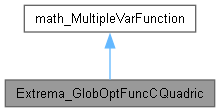This class implements function which calculate square Eucluidean distance between point on surface and nearest point on Conic. More...

`#include <Extrema_GlobOptFuncCQuadric.hxx>`[legend]

## Public Member Functions

Curve and surface should exist during all the lifetime of Extrema_GlobOptFuncCQuadric. More...

void LoadQuad (const Adaptor3d_Surface *S, const Standard_Real theUf, const Standard_Real theUl, const Standard_Real theVf, const Standard_Real theVl)

virtual Standard_Integer NbVariables () const
Returns the number of variables of the function. More...

virtual Standard_Boolean Value (const math_Vector &theX, Standard_Real &theF)
Computes the values of the Functions <F> for the variable <X>. returns True if the computation was done successfully, otherwise false. More...

void QuadricParameters (const math_Vector &theCT, math_Vector &theUV) const
Parameters of quadric for point on curve defined by theCT. More...Public Member Functions inherited from math_MultipleVarFunction
virtual Standard_Integer GetStateNumber ()
return the state of the function corresponding to the latestt call of any methods associated to the function. This function is called by each of the algorithms described later which define the function Integer Algorithm::StateNumber(). The algorithm has the responsibility to call this function when it has found a solution (i.e. a root or a minimum) and has to maintain the association between the solution found and this StateNumber. Byu default, this method returns 0 (which means for the algorithm: no state has been saved). It is the responsibility of the programmer to decide if he needs to save the current state of the function and to return an Integer that allows retrieval of the state. More...

virtual ~math_MultipleVarFunction ()

## Detailed Description

This class implements function which calculate square Eucluidean distance between point on surface and nearest point on Conic.

## ◆ NbVariables()

 virtual Standard_Integer Extrema_GlobOptFuncCQuadric::NbVariables ( ) const
virtual

Returns the number of variables of the function.

Implements math_MultipleVarFunction.

 void Extrema_GlobOptFuncCQuadric::QuadricParameters ( const math_Vector & theCT, math_Vector & theUV ) const

Parameters of quadric for point on curve defined by theCT.

## ◆ Value()

 virtual Standard_Boolean Extrema_GlobOptFuncCQuadric::Value ( const math_Vector & X, Standard_Real & F )
virtual

Computes the values of the Functions <F> for the variable <X>. returns True if the computation was done successfully, otherwise false.

Implements math_MultipleVarFunction.

The documentation for this class was generated from the following file: